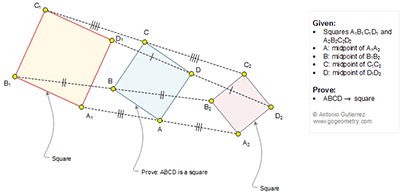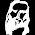Tuesday, August 4, 2015

Geometry Problem 1145: Three Squares, Midpoints

Geometry Problem
Level: Mathematics Education, High School, Honors Geometry, College.

Click the figure below to see the complete problem 1145.1.Let z(P) be the complex number representing P.

z(C₁)—z(B₁) = i [z(A₁)—z(B₁)]
z(C₂)—z(B₂) = i [z(A₂)—z(B₂)]
summing up, we have
z(C)—z(B) = i [z(A)—z(B)]

Thus, AB=BC and ∠ABC=90°.
Similar argument for others.

Hence, ABCD is a square.

2.Let O is the center of spiral similarity to transform square A1B1C1D1 to A2B2C2D2
We will have ∆A1OA2 ~ ∆C1OC2~ ∆B1OB2~ ∆D1OD2… (properties of spiral similarity transformation)
With medians OA, OB, OC, OD
Due to similarity of these triangles we also have
1. ∠ (A1OA)= ∠ (C1OC)= ∠ (B1OB)= ∠ (D1OD)=θ … (angles forms between corresponding sides to corresponding medians of similar triangles)
2. OA/OA1=OB/OB1=OC/OC1= OD/OD1=k …( ratio of corresponding medians to corresponding sides of similar triangles)
So square ABCD will be the image of square A1B1C1D1 in the spiral similarity transformation ( center O, angle of rotation= θ and factor of dilation = k)
Since A1B1C1D1 is a square so the image ABCD will be a square